Courses

# Flywheel And Governor - MCQ Test 2

## 30 Questions MCQ Test RRB JE for Mechanical Engineering | Flywheel And Governor - MCQ Test 2

Description
This mock test of Flywheel And Governor - MCQ Test 2 for Mechanical Engineering helps you for every Mechanical Engineering entrance exam. This contains 30 Multiple Choice Questions for Mechanical Engineering Flywheel And Governor - MCQ Test 2 (mcq) to study with solutions a complete question bank. The solved questions answers in this Flywheel And Governor - MCQ Test 2 quiz give you a good mix of easy questions and tough questions. Mechanical Engineering students definitely take this Flywheel And Governor - MCQ Test 2 exercise for a better result in the exam. You can find other Flywheel And Governor - MCQ Test 2 extra questions, long questions & short questions for Mechanical Engineering on EduRev as well by searching above.
QUESTION: 1

### The maximum fluctuation of energy Ef, during a cycle for a flywheel is (Where, I = Mass moment of inertia of the flywheel ωav = Average rotational speed Kes = Coefficient of fluctuation of speed)

Solution: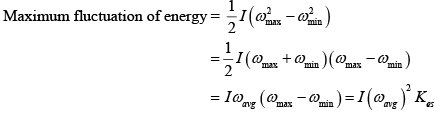QUESTION: 2

Solution:
QUESTION: 3

### In the case of a flywheel, the maximum fluctuation of energy is the

Solution:
QUESTION: 4

A spring controlled governor is found unstable. It can be made stable by

Solution:

A spring controlled governor can be made stable by decreasing the spring stiffness.

QUESTION: 5

Consider the following parameters:

1. Limit of peripheral speed
2. Limit of centrifugal stress
3. Coefficient of fluctuation of speed
4. Weight of the rim

Which of these parameters are used in the calculation of the diameter of fly wheel rim?

Solution:

Limit of centrifugal stress is not considered.

QUESTION: 6

Which one .of the following statement is correct?

A governor will be stable if the radius of rotation of the balls

Solution:
QUESTION: 7

What is the value of the radius of gyration of disc type flywheel as compared to rim type flywheel for the same diameter?

Solution: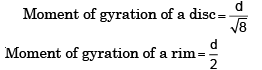QUESTION: 8

The sensitivity of an isochronous governor is

Solution: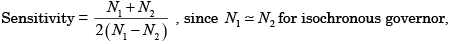sensitivity = α.

QUESTION: 9

For minimizing speed fluctuations of an engine as a prime mover, it must have

Solution:
QUESTION: 10

The nature of the governors is shown by the graph between radius (r) of rotation and controlling force (F). Which of the following is an isochronous governor?

Solution:
QUESTION: 11

Match List-I with List-II and select the correct answer using the codes given below the Lists: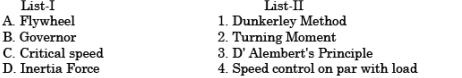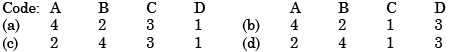Solution:
QUESTION: 12

A Hartnell governor has its controlling force F given by

F = p + qr
Where, is the radius of the balls and p and q are constants.
The governor becomes isochronous when

Solution:

For isochronous governor F = qr
So P should be zero and q be + ve.

QUESTION: 13

A flywheel is fitted to the crankshaft of an engine having 'E' amount of indicated work per revolution and permissible limits of co-efficient of fluctuation of energy and speed as Ke and Ks respectively.

The kinetic energy of the flywheel is then given by

Solution:
QUESTION: 14

Assertion (A): The degree of hunting with an unstable governor will be less than with an isochronous governor.

Reason (R): With an unstable governor, once the sleeve has moved from one extreme position to the other, a finite change of speed is required to cause it to move back again.

Solution:
QUESTION: 15

If the rotating mass of a rim type fly wheel is distributed on another rim type flywheel whose mean radius is half mean radius of the former, then energy stored in the latter at the same speed will be

Solution:

Energy stored ∞ Iω2 , also I∞k2 (k = radius of gyration which is function of radius of wheel)
:. If radius is reduced to half, then energy stored will be reduced to one-fourth.

QUESTION: 16

Consider the following statements:

1. The condition of stability of a governor requires that the slope of the controlling force curve should be less than that of the line representing the centripetal force at the equilibrium speed under consideration.
2. For a centrifugal governor when the load on the prime mover drops suddenly, the sleeve should at once reach the lower-most position.

Which of the statements given above is/are correct?

Solution:
QUESTION: 17

For the same indicated work per cycle, mean speed and permissible fluctuation of speed, what is the size of flywheel required for a multi-cylinder engine in comparison to a single cylinder engine?

Solution:
QUESTION: 18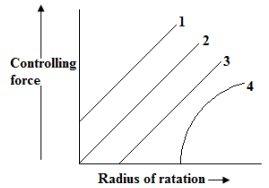The controlling force curves for a spring-controlled governor are shown in the above figure. Which curve represents a stable governor?

Solution:
QUESTION: 19

The turning moment diagram for a single cylinder double acting steam engine consists of +ve and –ve loops above and bellow the average torque line. For the +ve loop, the ratio of the speeds of the flywheel at the beginning and the end is which one of the following?

Solution: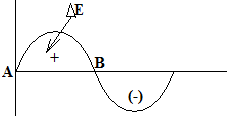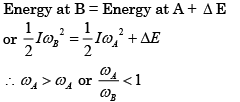QUESTION: 20

Consider the following statements concerning centrifugal governors:

1. The slope of the controlling force curve should be less than that of the straight line representing the centripetal force at the speed considered for the stability of a centrifugal governor.
2. Isochronism for a centrifugal governor can be achieved only at the expense of stability.
3. When sleeve of a centrifugal governor reaches its topmost position, the engine should develop maximum power.

Which of the statements given above is/are correct?

Solution:
QUESTION: 21

A certain machine requires a torque of (500 + 50Sinθ) KNm to derive it, θ where is the angle of rotation of shaft measured from certain datum. The machine is directly coupled to an engine which produces a toques (500 +50sin θ) KNm in a cycle how many times the value of torque of machine and engine will be identical

Solution: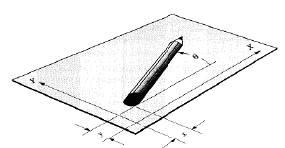QUESTION: 22

The height of a simple Watt governor running at a speed ‘N’ is proportional to

Solution: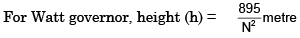QUESTION: 23

In which of the following case, the turning moment diagram will have least variations:

Solution:
QUESTION: 24

The height of Watt's governor is

Solution:
QUESTION: 25

The given figure shows the output torque plotted against crank positions for a single cylinder fourstroke-cycle engine. The areas lying above the zero-torque line represent positive work and the areas below represent negative work. The engine drives a machine which offers a resisting torque equal to the average torque. The relative magnitudes of the hatched areas are given by the numbers (in the areas) as shown: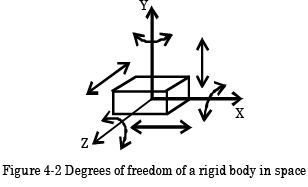During the cycle, the minimum speed occurs in the engine at

Solution:

Minimum speed occurs at point where cumulative torque is least, i.e. -23 at F.

QUESTION: 26

The plots of controlling force versus radii of rotation of the balls of spring controlled governors are shown in the given diagram. A stable governor is characterised by the curve labelled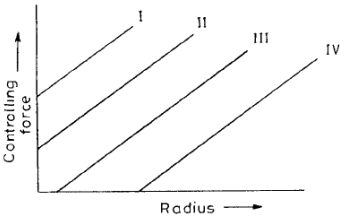Solution:

For stable governor, F = qr - p which is possible with curve IV.

QUESTION: 27

In a four stroke I.C. engine, the turning moment during the compression stroke is

Solution:
QUESTION: 28

For a spring controlled governor to be stable, the controlling force (F) is related to the radius (r) by the equation

Solution:
QUESTION: 29

The amount of energy absorbed by a flywheel is determined from the

Solution:
QUESTION: 30

The sensitivity dh/dN of a given Porter Governor. Where 'h' is the height of the pin point A from the sleeve and N is the ripe. m., is proportional to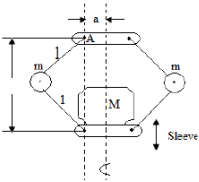Solution: